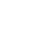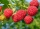# Least common denominator calculator

### What is LCD?

The lowest common denominator or least common denominator (abbreviated LCD) is the least common multiple of the denominators of a set of fractions. It is the smallest positive integer that is a multiple of each denominator in the set. Fractions write with fraction bar / like 3/4 .

Example: for LCD calculation of three fractions 1/2 2/3 5/4, enter 1/2 2/3 5/4.

### How to calculate LCD

The same fraction can be expressed in many different forms. If the ratio between numerator and denominator is the same, the fractions represent the same rational number.

The least common denominator of a set of fractions is the least number that is a multiple of all the denominators: their "least common multiple".
The Method of calculating the LCD is the same as the calculation least common multiple of fractions denominators.
The simple method for common computing denominators (not least at all) is to multiply all denominators.

## Questions and word problems to LCD

• LCD 2The least common denominator of 2/5, 1/2, and 3/4
• FractionsSort fractions z1 = (6)/(11); z2 = (10)/(21); z3 = (19)/(22) by its size. Result write as three serial numbers 1,2,3.
• Two gearsA large gear will be used to turn a smaller gear. The large gear will make 75 revolutions per minute. The smaller gear must make 384 revolutions per minute. Find the smallest number of teeth each gear could have. [Hint: Use either GCF or LCM. ]
• LCD two fractionsWhat is the least common denominator of 11/15 and 12/19?On the meadow grazing horses, cows, and sheep, together with less than 200. If cows were 45 times more, horses 60 times more, and sheep 35 times more than there are now, their numbers would equally. How many horses, cows, and sheep are on the meadow togetAlena collected 7.8 kg of blueberries, 2.6 kg of blackberries, and 3.9 kg of cranberries. Express the ratio in the smallest natural numbers in this order.There are given numbers A = 135, B = 315. Find the smallest natural number R greater than 1 so that the proportions R:A, R:B are with the remainder 1.